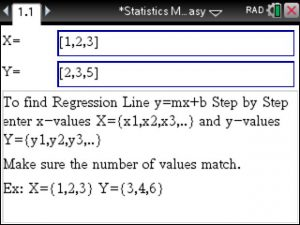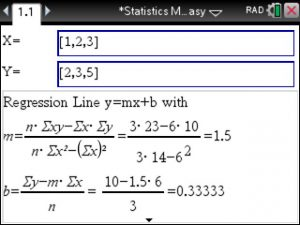# Correlation Coefficient and Coefficient of Determination using the TiNSpire – Step by Step

Linear Regression you just type in the given x and y values. Next the linear regression is computed for you along with the  Correlation Coefficient and the Coefficient of Determination.

That simple.Posted on Categories statistics, tinspirecx